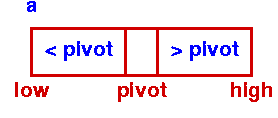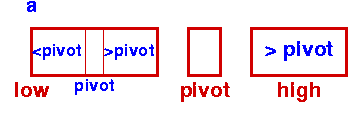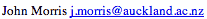Data Structures and Algorithms 7.3 Quick Sort

Quicksort is a very efficient sorting algorithm invented by C.A.R. Hoare. It has two phases:

• the partition phase and
• the sort phase.
As we will see, most of the work is done in the partition phase - it works out where to divide the work. The sort phase simply sorts the two smaller problems that are generated in the partition phase.

This makes Quicksort a good example of the divide and conquer strategy for solving problems. (You've already seen an example of this approach in the binary search procedure.) In quicksort, we divide the array of items to be sorted into two partitions and then call the quicksort procedure recursively to sort the two partitions, ie we divide the problem into two smaller ones and conquer by solving the smaller ones. Thus the conquer part of the quicksort routine looks like this:
 ```quicksort( void *a, int low, int high ) { int pivot; /* Termination condition! */ if ( high > low ) { pivot = partition( a, low, high ); quicksort( a, low, pivot-1 ); quicksort( a, pivot+1, high ); } } ```Initial Step - First PartitionSort Left Partition in the same way
For the strategy to be effective, the partition phase must ensure that all the items in one part (the lower part) and less than all those in the other (upper) part.

To do this, we choose a pivot element and arrange that all the items in the lower part are less than the pivot and all those in the upper part greater than it. In the most general case, we don't know anything about the items to be sorted, so that any choice of the pivot element will do - the first element is a convenient one.

As an illustration of this idea, you can view this animation, which shows a partition algorithm in which items to be sorted are copied from the original array to a new one: items smaller than the pivot are placed to the left of the new array and items greater than the pivot are placed on the right. In the final step, the pivot is dropped into the remaining slot in the middle.
 QuickSort Animation This animation was based on a suggestion made by Jeff Rohl; it was written by Woi Ang.
Observe that the animation uses two arrays for the items being sorted: thus it requires O(n) additional space to operate. However, it's possible to partition the array in place. The next page shows a conventional implementation of the partition phase which swaps elements in the same array and thus avoids using extra space.

### Key terms

Divide and Conquer Algorithms
Algorithms that solve (conquer) problems by dividing them into smaller sub-problems until the problem is so small that it is trivially solved.
in place
In place sorting algorithms don't require additional temporary space to store elements as they sort; they use the space originally occupied by the elements.

©, 1998